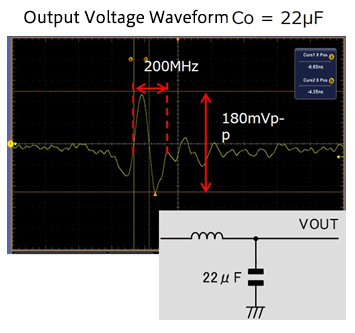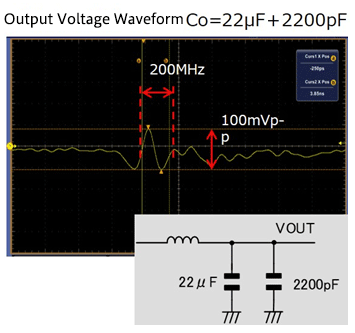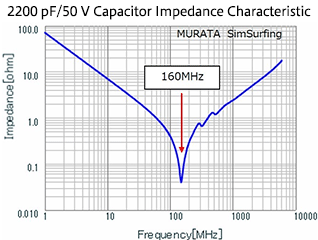Technical Information Site of Power Supply Design

2018.10.25 Switching Noise - EMC

# Measures to Address Noise Using Capacitors

Dealing with Noise Using Capacitors

In the previous article, the frequency characteristics of capacitors were explained. This time, a summary of measures to address noise using the capacitors discussed here is given, together with related concepts.

Measures to Address Noise Using Capacitors

There are various types of noise, having a wide variety of characteristics. Hence there are also various noise countermeasures, that is, methods for reducing noise. Here we are essentially considering noise relating to switching power supplies, and so the reader should assume high-frequency noise with comparatively low voltage levels, present in DC voltages. Moreover, in addition to capacitors, Zener diodes, suppressors for noise, surge or ESD and the like are also used to deal with noise. There are various components to deal with noise according to the noise properties; when DC/DC converters are assumed, due to the circuits and voltage levels involved, in many cases LCR circuits are used.

Concepts Underlying Noise Countermeasures Using Capacitors

Below is an example in which the noise in the output voltage of a DC/DC converter is suppressed through addition of capacitors.The waveform on the left is for a case in which the capacitor of the output LC filter is 22 μF; there is noise (ringing, reflection) at about 200 MHz with an amplitude of 180 mVp-p. In order to reduce this noise, a 2200 pF capacitor was added; the result is the waveform on the right. By adding the 2200 pF capacitor, the noise was attenuated to about 100 mV.

What the reader should be asking here is, "why 2200 pF?" The frequency characteristic of the impedance of the added capacitor is shown below.

The impedance is lowest near 160 MHz; the reason for selecting this 2200 pF capacitor was to utilize this impedance characteristic in order to attenuate the noise amplitude at about 2 MHz.

In this approach, by adding the capacitor so as to lower the impedance at the frequency of the noise being targeted, the noise amplitude is reduced.

When thus adding a capacitor to reduce noise, it is necessary to ascertain the frequency of the noise (ringing, reflection), and then select a capacitor having a corresponding impedance frequency characteristic.In this article, we have given a summary explanation of noise countermeasures using capacitors. Next time, we will explain the use of effective decoupling capacitors.

#### Key Points:

・Noise amplitudes can be reduced by lowering the impedance at the frequency of the targeted noise.

・A capacitor to be used to address noise is selected for its impedance frequency characteristic rather than for its capacitance value.